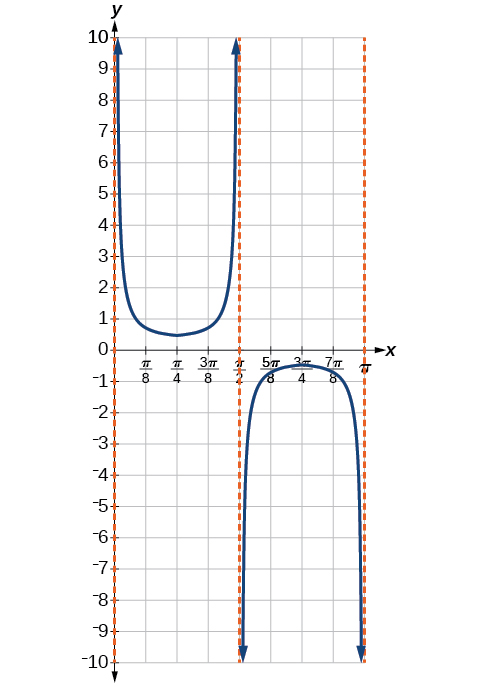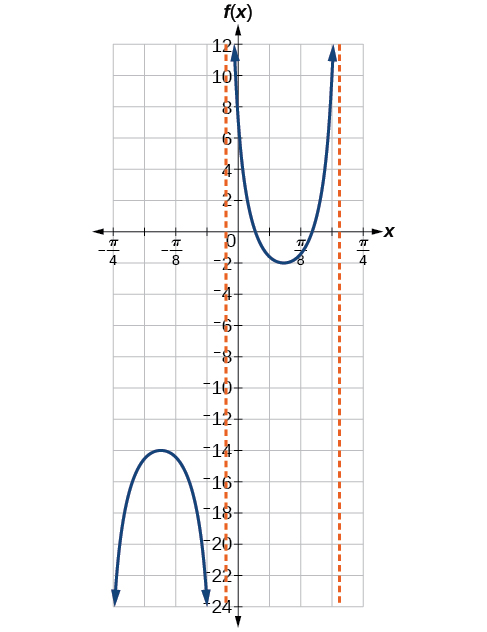# 2.2 Graphs of the other trigonometric functions  (Page 5/9)

 Page 5 / 9
$y=A\mathrm{sec}\left(Bx-C\right)+D$
$y=A\mathrm{csc}\left(Bx-C\right)+D$

## Features of the graph of y = A Sec( Bx − C )+ D

• The stretching factor is $\text{\hspace{0.17em}}|A|.$
• The period is $\text{\hspace{0.17em}}\frac{2\pi }{|B|}.$
• The domain is $\text{\hspace{0.17em}}x\ne \frac{C}{B}+\frac{\pi }{2|B|}k,$ where $\text{\hspace{0.17em}}k\text{\hspace{0.17em}}$ is an odd integer.
• The range is $\text{\hspace{0.17em}}\left(-\infty ,-|A|\right]\cup \left[|A|,\infty \right).$
• The vertical asymptotes occur at $\text{\hspace{0.17em}}x=\frac{C}{B}+\frac{\pi }{2|B|}k,$ where $\text{\hspace{0.17em}}k\text{\hspace{0.17em}}$ is an odd integer.
• There is no amplitude.
• $y=A\mathrm{sec}\left(Bx\right)\text{\hspace{0.17em}}$ is an even function because cosine is an even function.

## Features of the graph of y = A Csc( Bx − C )+ D

• The stretching factor is $\text{\hspace{0.17em}}|A|.$
• The period is $\text{\hspace{0.17em}}\frac{2\pi }{|B|}.$
• The domain is $\text{\hspace{0.17em}}x\ne \frac{C}{B}+\frac{\pi }{2|B|}k,$ where $\text{\hspace{0.17em}}k\text{\hspace{0.17em}}$ is an integer.
• The range is $\text{\hspace{0.17em}}\left(-\infty ,-|A|\right]\cup \left[|A|,\infty \right).$
• The vertical asymptotes occur at $\text{\hspace{0.17em}}x=\frac{C}{B}+\frac{\pi }{|B|}k,$ where $\text{\hspace{0.17em}}k\text{\hspace{0.17em}}$ is an integer.
• There is no amplitude.
• $y=A\mathrm{csc}\left(Bx\right)\text{\hspace{0.17em}}$ is an odd function because sine is an odd function.

Given a function of the form $\text{\hspace{0.17em}}y=A\mathrm{sec}\left(Bx\right),\text{\hspace{0.17em}}$ graph one period.

1. Express the function given in the form $\text{\hspace{0.17em}}y=A\mathrm{sec}\left(Bx\right).$
2. Identify the stretching/compressing factor, $\text{\hspace{0.17em}}|A|.$
3. Identify $\text{\hspace{0.17em}}B\text{\hspace{0.17em}}$ and determine the period, $\text{\hspace{0.17em}}P=\frac{2\pi }{|B|}.$
4. Sketch the graph of $\text{\hspace{0.17em}}y=A\mathrm{cos}\left(Bx\right).$
5. Use the reciprocal relationship between $\text{\hspace{0.17em}}y=\mathrm{cos}\text{\hspace{0.17em}}x\text{\hspace{0.17em}}$ and $\text{\hspace{0.17em}}y=\mathrm{sec}\text{\hspace{0.17em}}x\text{\hspace{0.17em}}$ to draw the graph of $\text{\hspace{0.17em}}y=A\mathrm{sec}\left(Bx\right).$
6. Sketch the asymptotes.
7. Plot any two reference points and draw the graph through these points.

## Graphing a variation of the secant function

Graph one period of $\text{\hspace{0.17em}}f\left(x\right)=2.5\mathrm{sec}\left(0.4x\right).$

• Step 1. The given function is already written in the general form, $\text{\hspace{0.17em}}y=A\mathrm{sec}\left(Bx\right).$
• Step 2. $\text{\hspace{0.17em}}A=2.5\text{\hspace{0.17em}}$ so the stretching factor is $\text{\hspace{0.17em}}\text{2}\text{.5}\text{.}$
• Step 3. $\text{\hspace{0.17em}}B=0.4\text{\hspace{0.17em}}$ so $\text{\hspace{0.17em}}P=\frac{2\pi }{0.4}=5\pi .\text{\hspace{0.17em}}$ The period is $\text{\hspace{0.17em}}5\pi \text{\hspace{0.17em}}$ units.
• Step 4. Sketch the graph of the function $\text{\hspace{0.17em}}g\left(x\right)=2.5\mathrm{cos}\left(0.4x\right).$
• Step 5. Use the reciprocal relationship of the cosine and secant functions to draw the cosecant function.
• Steps 6–7. Sketch two asymptotes at $\text{\hspace{0.17em}}x=1.25\pi \text{\hspace{0.17em}}$ and $\text{\hspace{0.17em}}x=3.75\pi .\text{\hspace{0.17em}}$ We can use two reference points, the local minimum at $\text{\hspace{0.17em}}\left(0,2.5\right)\text{\hspace{0.17em}}$ and the local maximum at $\text{\hspace{0.17em}}\left(2.5\pi ,-2.5\right).\text{\hspace{0.17em}}$ [link] shows the graph.

Graph one period of $\text{\hspace{0.17em}}f\left(x\right)=-2.5\mathrm{sec}\left(0.4x\right).$

This is a vertical reflection of the preceding graph because $\text{\hspace{0.17em}}A\text{\hspace{0.17em}}$ is negative.Do the vertical shift and stretch/compression affect the secant’s range?

Yes. The range of $\text{\hspace{0.17em}}f\left(x\right)=A\mathrm{sec}\left(Bx-C\right)+D\text{\hspace{0.17em}}$ is $\left(-\infty ,-|A|+D\right]\cup \left[|A|+D,\infty \right).$

Given a function of the form $\text{\hspace{0.17em}}f\left(x\right)=A\mathrm{sec}\left(Bx-C\right)+D,\text{\hspace{0.17em}}$ graph one period.

1. Express the function given in the form $\text{\hspace{0.17em}}y=A\text{\hspace{0.17em}}\mathrm{sec}\left(Bx-C\right)+D.$
2. Identify the stretching/compressing factor, $\text{\hspace{0.17em}}|A|.$
3. Identify $\text{\hspace{0.17em}}B\text{\hspace{0.17em}}$ and determine the period, $\text{\hspace{0.17em}}\frac{2\pi }{|B|}.$
4. Identify $\text{\hspace{0.17em}}C\text{\hspace{0.17em}}$ and determine the phase shift, $\text{\hspace{0.17em}}\frac{C}{B}.$
5. Draw the graph of $\text{\hspace{0.17em}}y=A\text{\hspace{0.17em}}\mathrm{sec}\left(Bx\right)\text{\hspace{0.17em}}.$ but shift it to the right by $\text{\hspace{0.17em}}\frac{C}{B}\text{\hspace{0.17em}}$ and up by $\text{\hspace{0.17em}}D.$
6. Sketch the vertical asymptotes, which occur at $\text{\hspace{0.17em}}x=\frac{C}{B}+\frac{\pi }{2|B|}k,$ where $\text{\hspace{0.17em}}k\text{\hspace{0.17em}}$ is an odd integer.

## Graphing a variation of the secant function

Graph one period of $\text{\hspace{0.17em}}y=4\mathrm{sec}\left(\frac{\pi }{3}x-\frac{\pi }{2}\right)+1.$

• Step 1. Express the function given in the form $\text{\hspace{0.17em}}y=4\mathrm{sec}\left(\frac{\pi }{3}x-\frac{\pi }{2}\right)+1.$
• Step 2. The stretching/compressing factor is $|A|=4.$
• Step 3. The period is
• Step 4. The phase shift is
• Step 5. Draw the graph of $\text{\hspace{0.17em}}y=A\mathrm{sec}\left(Bx\right),$ but shift it to the right by $\text{\hspace{0.17em}}\frac{C}{B}=1.5\text{\hspace{0.17em}}$ and up by $\text{\hspace{0.17em}}D=6.$
• Step 6. Sketch the vertical asymptotes, which occur at $\text{\hspace{0.17em}}x=0,x=3,$ and $\text{\hspace{0.17em}}x=6.\text{\hspace{0.17em}}$ There is a local minimum at $\text{\hspace{0.17em}}\left(1.5,5\right)\text{\hspace{0.17em}}$ and a local maximum at $\text{\hspace{0.17em}}\left(4.5,-3\right).\text{\hspace{0.17em}}$ [link] shows the graph.

Graph one period of $\text{\hspace{0.17em}}f\left(x\right)=-6\mathrm{sec}\left(4x+2\right)-8.$where we get a research paper on Nano chemistry....?
nanopartical of organic/inorganic / physical chemistry , pdf / thesis / review
Ali
what are the products of Nano chemistry?
There are lots of products of nano chemistry... Like nano coatings.....carbon fiber.. And lots of others..
learn
Even nanotechnology is pretty much all about chemistry... Its the chemistry on quantum or atomic level
learn
da
no nanotechnology is also a part of physics and maths it requires angle formulas and some pressure regarding concepts
Bhagvanji
hey
Giriraj
Preparation and Applications of Nanomaterial for Drug Delivery
revolt
da
Application of nanotechnology in medicine
what is variations in raman spectra for nanomaterials
I only see partial conversation and what's the question here!
what about nanotechnology for water purification
please someone correct me if I'm wrong but I think one can use nanoparticles, specially silver nanoparticles for water treatment.
Damian
yes that's correct
Professor
I think
Professor
Nasa has use it in the 60's, copper as water purification in the moon travel.
Alexandre
nanocopper obvius
Alexandre
what is the stm
is there industrial application of fullrenes. What is the method to prepare fullrene on large scale.?
Rafiq
industrial application...? mmm I think on the medical side as drug carrier, but you should go deeper on your research, I may be wrong
Damian
How we are making nano material?
what is a peer
What is meant by 'nano scale'?
What is STMs full form?
LITNING
scanning tunneling microscope
Sahil
how nano science is used for hydrophobicity
Santosh
Do u think that Graphene and Fullrene fiber can be used to make Air Plane body structure the lightest and strongest. Rafiq
Rafiq
what is differents between GO and RGO?
Mahi
what is simplest way to understand the applications of nano robots used to detect the cancer affected cell of human body.? How this robot is carried to required site of body cell.? what will be the carrier material and how can be detected that correct delivery of drug is done Rafiq
Rafiq
if virus is killing to make ARTIFICIAL DNA OF GRAPHENE FOR KILLED THE VIRUS .THIS IS OUR ASSUMPTION
Anam
analytical skills graphene is prepared to kill any type viruses .
Anam
Any one who tell me about Preparation and application of Nanomaterial for drug Delivery
Hafiz
what is Nano technology ?
write examples of Nano molecule?
Bob
The nanotechnology is as new science, to scale nanometric
brayan
nanotechnology is the study, desing, synthesis, manipulation and application of materials and functional systems through control of matter at nanoscale
Damian
Is there any normative that regulates the use of silver nanoparticles?
what king of growth are you checking .?
Renato
What fields keep nano created devices from performing or assimulating ? Magnetic fields ? Are do they assimilate ?
why we need to study biomolecules, molecular biology in nanotechnology?
?
Kyle
yes I'm doing my masters in nanotechnology, we are being studying all these domains as well..
why?
what school?
Kyle
biomolecules are e building blocks of every organics and inorganic materials.
Joe
how did you get the value of 2000N.What calculations are needed to arrive at it
Privacy Information Security Software Version 1.1a
Good
Got questions? Join the online conversation and get instant answers!By Steve GibbsBy Dan ArielyBy Laurence BailenBy OpenStaxBy Tess ArmstrongBy OpenStaxBy Katie MontroseBy Angela EckmanBy Madison ChristianBy OpenStax